Skip to content

# Ways to Improve the EDS Quantitative Results Accuracy: Efficiency and eZAF SCC Database

### Introduction

The definition of the “Quant” task is that it simply converts the elemental characteristic X-rays into weight fractions, showing the concentrations of the elements. The element-related signal must be extracted from raw measured data, e.g., from the spectrum. Some processing and pre-evaluation steps are required before obtaining the pure signal, e.g., corrected with regard to detector artifacts, to determine the background (mainly the bremsstrahlung), and if necessary, also to deconvolute different element origin data if it is not possible to get separate measured data due to limited spectrometer resolution (peak deconvolution). With its state-of-the-art algorithm, the EDAX spectra evaluation software is equipped to handle all parts of the spectra processing to get the best determination of the pure-element signals extracted from spectra.

### Results and Discussion

The used signals are normally:

• Net-counts of characteristic X-rays (means bremsstrahlung background is already subtracted) for standardless evaluation
• P/B-ratios, which are net-counts of characteristic X-rays divided by measured bremsstrahlung of the same energy, for standardless evaluation
• K-ratios, which are net-count ratios of characteristic X-rays of an unknown specimen measurement divided by the measurements of the net-counts from one or several standard(s) with known element composition, for standards-based evaluation

The measured elemental signals provide the raw data for the quantification algorithm. Firstly, they depend on the excitation, the primary electron energy, which is determined by the high voltage (HV) of the electron microscope. This is the Generation of X-rays that depends on the concentration of the element, which is the base analytical relation.

Net-counts = X-ray Generation (C, Z, E) * Absorption (Z, Zm, E) * Fluorescence (Z, Zm, E) * Efficiency (E)

Z is the atomic number of the considered element; E is the energy of the element-line used for Quant; C is the concentration of the element, and Zm stands for all other elements in the composition that have an effect depending on their concentrations Cm.

It also depends on the other elements in the sample and the entire composition via self-absorption of generated X-rays in the sample (Absorption) and enhancement of the considered X-rays by additional fluorescence effects (Fluorescence). Therefore, the measured signals need to be corrected with regards to the different physical effects, Absorption, and Fluorescence. This process is named ‘matrix-correction’ and depends on the composition of the matrix-elements (Zm). The quantification algorithm (Quant) needs to work iteratively because the required knowledge about the composition of all other elements is also still not known.

The significant effects are Generation and Absorption. Fluorescence is an enhanced effect of additional X-rays that were not generated by the primary electrons. This can be important with low-concentration elements and special constellations of major elements in the composition. But it is usually a minor effect, affecting only the analytics if element concentrations are minimal in special cases. Therefore, it will not be discussed here with the topic of general Quant accuracy improvement.

It is a basic thought that if an element in a specimen has double the counts compared to another specimen, then one would assume that the concentration of this element is double as well. It is almost true in terms of the Generation of X-rays for a given HV. But unfortunately, this is usually not always true with Energy Dispersive Spectroscopy (EDS) in a Scanning Electron Microscope (SEM), which is a highly non-linear analytical method. The Absorption of generated X-rays in a specimen does influence the measured results. It is even more uncertain in cases where the effect is high because Mass Absorption Coefficients (MACs) have jumps and determine the properties for absorption physics in a specimen. They are especially uncertain with X-ray energies close to the electron shell energies (the jumps) of the elements in a specimen’s composition. One example is given in Figure 1. A simulated binary example of an Al/Si compound, whereby the Si radiation has a high absorption effect of Al with non-linear behavior. But it is not the case for measurements with PeBaZAF, which use P/B-ratios with Si, which are still quite linear.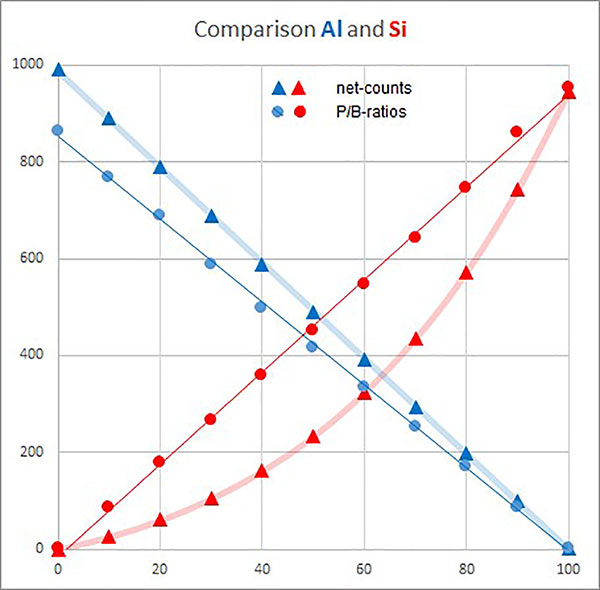Figure 1. Measured X-ray net-counts vs. P/B values with a calculated Al/Si binary sample (Al blue; Si red). The x-axis shows the element concentrations of Si or Al, and the y-axis depicts the P/B and net-counts arbitrary units.

The P/B is like linearizing the Quant question. But it needs to be mentioned that the problem is partially delegated to determine the P/B measurement values, which are interpolations from the real measured spectrum. This is because the pure bremsstrahlung measured counts of the same energy are not measurable directly at the same energy where characteristic lines are. With modern Silicon Drift Detectors (SDDs) that measure high count rates, there is no longer a statistic limitation of the P/B signal . It is more the limiting systematic error with the determination of divided bremsstrahlung-background in relation to P/B. However, the uncertainty associated with determining the P/B values is not as high as with the absorption effects that accompany net-count-based measurements.

Figure 2 shows the substantial absorption effects of Si-K radiation in an Al-rich specimen composition. The effects are not only visible in the bremsstrahlung calculated absorption jumps, but the peak heights (net-counts) of both elements are much different, even though both have the same concentration in the specimen.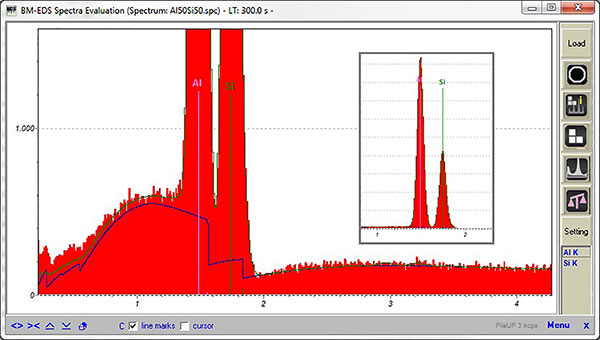Figure 2. A simulated spectrum example with 50% mass fraction of both elements (often named weight%) at 20 kV excitation, evaluated with the EDAX advanced spectra evaluation software tool.

Everything with Quant deals with the specimen emitted X-rays, not with the measured net-counts:

Net-counts (emitted) = Net-counts (measured) / Efficiency (E)

So, the first task is to calculate the true emitted X-rays using the required knowledge associated with detector efficiency. But this is not always required. The efficiency cancels out with a standards comparison measurement with FSQ (Full Standards Quant) if for each unknown element, a standard was measured with the same detector and geometry. The analytical results with standardless P/B-based quantification are also not influenced. But because the PeBaZAF is working only for energies >1 keV P/B-based quantification, it is still required to consider the detector efficiency with Z < 11 elements, where the net-counts are used.

Therefore, the PeBaZAF standardless method achieves about ±10% relative result accuracy (standard deviation 5%) even without the need for any additional use of an empirically measured database [1,2]. It is because the Quant question is partially linearized, and all Z ≥ 11 element determinations are not influenced by detector efficiency, which is the next point of uncertainty to be discussed.

The detector efficiency is quite complex and sensitive, which influences all analytical results if an efficiency independent method is not used (such as FSQ with measurement of standards with the same system; like PeBaZAF all elements Z ≥11). There can be uncertainties with the detector and window-specific properties, and each individual detector has residual uncertainties due to manufacturing reasons, SEM geometry, aging, and window contaminations.

Knowledge about efficiency is required, especially for eZAF standardless, and FSQ if a standards data library must be created for use by other measurement systems without remeasurement of the standards.

For this reason, an Efficiency Correction Factor (ECF) can be determined and used empirically. It can provide efficiency corrections to consider the actual properties of used detectors .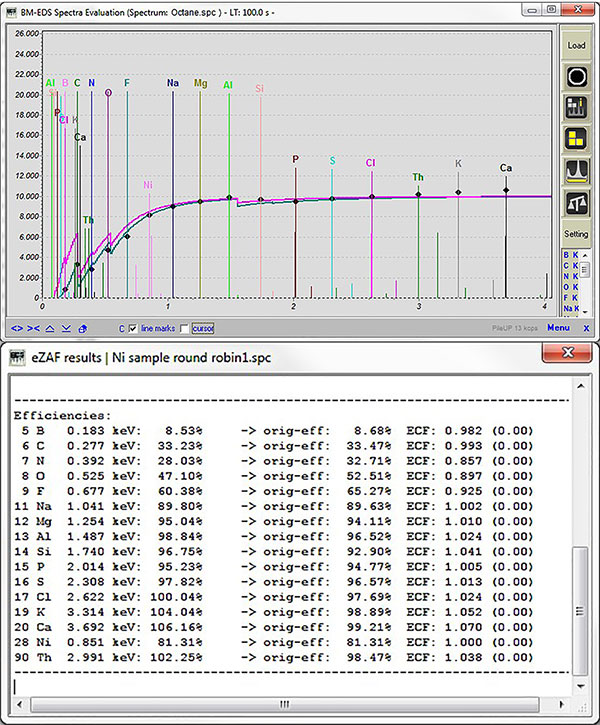Figure 3. a) Efficiency curve of older EDAX detectors (the pink line is window transmission; the green line is complete detector efficiency) with diamonds representing the true used efficiency by Quant, which can differ from theory and Synchrotron measured values by using ECFs. The example shows Quant internally applied ECFs to address a divergence effect by Moxtek windows lamella . b) Originally calculated efficiency (curve) corrected by ECF values.

In addition, the generation of X-rays already has uncertainties in Quant models and fundamental atomic data. It is more uncertain the closer the primary electron energy is to the excited element shell energy. We have introduced an empirical database, Standards Customized Coefficients (SCC) for eZAF standardless, to optimize the model and parameters for a given set of conditions.

Net-counts = X-ray Generation * SCC * Absorption * Fluorescence * Efficiency (E) * ECF

The detector-based effects on measured X-rays (possible to correct with ECF) must be separated from the specimen’s internal generation of X-rays (SCC). The ECF Efficiency correction values should be independent of the model, eZAF, or PeBaZAF (Z < 11). The SCC directly adjusts the X-ray Generation physics. Applying SCC factors makes it possible to compensate for general deviations in X-ray yields for given elements over a wide range of electron excitation energies . But it is also possible to improve the eZAF for applications where SCC values are measured empirically to compensate for standardless result deviations. But because this is just a factor, it cannot address non-linearities due to huge interdependencies between elements, as shown in Figure 1. However, following the “customized-standardless” method is quite useful for special limited application purposes and dedicated specimen composition ranges. One can generate (measure) a dedicated SCC dataset with known composition samples, making it possible to store this empirical dataset with a dedicated name. These created datasets can be used at any time for standardless eZAF if similar samples are measured again.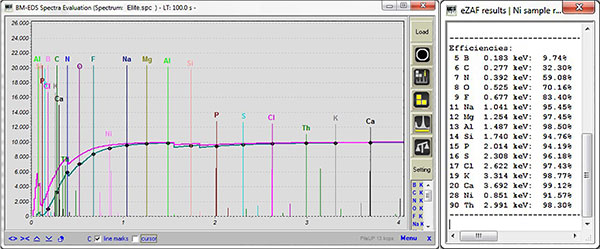Figure 4. Efficiency curve of more recent EDAX detectors (the pink line is window transmission; the green line is complete detector efficiency) with diamonds representing the true used efficiency by Quant. The theory and Synchrotron measured values are the same, and no divergence effect is visible with the new C2 type window grids.

For assessment and SCC determinations, it is compulsory to work with eZAF unnormalized results. Any normalization makes it hard to detect where the deviations might be from. In the Al/Si example, the challenging element is not the Al; it is entirely the Si in this specimen type. One cannot determine if the eZAF results are monitored continuously when normalized to 100%.

Also, the incorrect determination of Al is due to the incorrect Si results due to the normalization equation effect. And even the Si mistakes will be smaller; they will not be detectable with real deviations. To get eZAF not normalized results, a “reference measurement” is required with a pure element specimen. This means that this obtains the unknown absolute relation of eZAF to calculate absolute results, by adjusting this way the beam-current and/or detector solid angle. With routine analysis, eZAF should only be applied not-normalized to identify bad analysis results and to avoid analytical result blunders. And it is recommended to determine the case in which the analysis goes out of the reasonability via concentration total. In this instance, a new reference measurement should be taken. If everything is OK, one can use the normalized results for reporting and final analytical assessment. The reference value is the absolute adjustment of the measurement and, therefore, a core analytical parameter for eZAF, stored with each spectrum, that is just as important as HV, acquisition live-time, and count rate. The “reference measurement” is the key to improving eZAF and getting an absolute view.

It is impossible to address huge non-linear effects over big composition differences with a simple SCC factor for the element line series. The following example with an SCC-based Quant adjustment shows the limitations. The results are improved only for a specimen type and specific area of concentrations and compositions.

Figure 5a shows the eZAF calculated element compositions (unnormalized) for Al and Si over the Si content. The broad light-red line shows the original measurement effect (net-counts) for comparison. Therefore, the eZAF correction algorithm already did a great job. However, there are still deviations, especially with low Si concentrations, if X-rays are greatly absorbed by the dominating Al in the specimen.

The Si result deviations are still more than 20% relative to the Si compositions, up to 30% concentration. Figure 5b shows the same situation, but Si’s SCC value was determined using the 10% Si spectrum. The relative deviations for Si are now < 10% relative to all Quant results, up to a Si concentration of 30%. The problem with this is that the deviations exceed 30% relatively for high Si concentrations. The created empirical database (in this example, only one SCC value for Si-K) is not possible to use in general, but it is good to use it with Al/Si samples of low Si concentrations. One can name this special database “Si in high concentration Al” and store it, ready to use for similar specimen and applications. But once again, this is not recommended to use in a general context to improve eZAF in an empirical way; it would destroy all other analytical results for Si if Si is in high concentrations and if no Al is in the specimen. With this example, the pure Si result rises to more than 130%. Sure, if this result is normalized, it would be 100% if Al concentration is determined to be minimal.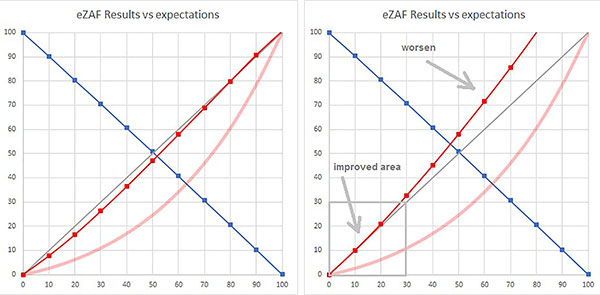Figure 5. a) Calculated concentration results by eZAF for binary Al/Si example specimen (blue Al; red Si) over the Si nominal concentration, all in % units. The broad light-red line is the Si net-count raw data curve from Figure 1, arbitrary units, not yet ZAF corrected. b) The same sample but with low Si concentrations using SCC.

But it matters in almost all other normalized cases because the element atomic-shell excitation relations to each other would get out of balance. Therefore, do not normalize your eZAF results all the time. Be aware that pure element specimen results will be corrupted and are only possible to watch with no normalization.

### Conclusion

The SCC database is dedicated to correct/adjust the excitation part of specimen physics with eZAF. It was required to assist with the standardless unnormalized calculation option. Only a reference measurement with one pure element specimen is required. It is only necessary to repeat if the conditions have been changed (e.g., the beam-current changed and is not under precise measurement control).

• To correct/adjust the element’s X-ray lines excitation in relation to each other (cross-sections of shell excitation and X-ray emission probabilities).
• To get similar results over a wide range of used HV (primarily electron energies).
• To improve results, “customized standardless” can be used for dedicated applications (e.g., for a defined specimen and/or composition type) because it can change the calculation in a manner that makes the accuracy of the same elements with entirely different concentrations or in completely different matrix composition worse with Quant.

The software operator and the analyst can access the SCC database. Improved eZAF results with “customized standardless” can be achieved with dedicated applications on your work. Additionally, there will be a factory all-purpose database available in the future.

It is the first step to achieve the focused goals for eZAF (Figure 6).Figure 6. The goal of improving eZAF accuracy with empirical measurements and databases [1,2].

### References

 Eggert F (2020) “Effect of the Silicon Drift Detector on EDAX Standardless Quant Methods” Microscopy Today 28/2 34-39
 EDAX-Insight (2019) “EDAX Standardless Quant Methods” 17/1 1-3
 Eggert F et al. (2021) “The Detector Efficiency Question with EDS” Microscopy and Microanalysis 27(S1) 1674-1676
 Rafaelson J, Eggert F, Kawabata M (2021) “EDS Quantification Using Fe L Peaks and Low Beam Energy” Microscopy and Microanalysis 27(S1) 1670-1672